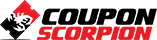[82% Off] Corporate Finance #4 Leverage & Break-Even Analysis

Learn about leverage and break-even analysis from a certified public accountant (CPA)

What you’ll learn

• Be able to explain the concept of leverage and why it is important
• Be able to use break even analysis for decision making
• Explain the concepts of degree of operating leverage, financial leverage, & combined leverage
• Calculate the break even point
• Calculate the cash break even point
• Use nonlinear breakeven analysis

Requirements

• A general understanding of financial concepts

Description

In this course we will cover the concepts of leverage and break-even analysis from a corporate finance perspective.

Leverage is a critical component of decision making in corporate finance and is an area of debate as to the optimal amount of leverage. Finding the best level of leverage may allow a business to grow much faster.

We will discuss the concepts of operating leverage, financial leverage, and combined leverage and include practical practice problems related to these critical concepts.

Break-even analysis is a fundamental tool in decision making. Learners will understand the concept of break-even analysis, including the tool of breaking costs up by their behavior, labeling them fixed costs and variable costs. Labeling costs by category of fixed and variable can seem unnatural at first, because we often label costs by function, what the cost is designed to do, like operating expenses and cost of goods sold. Labeling cost by behavior helps us see how the cost changes with relation to increases in production level, a useful concept for projecting into the future.

The course will show how to calculate the break-even point and the cash break-even point. We will also discuss the concept of nonlinear break-even analysis.

This course will include many practice problems, often including downloadable Excel worksheets. Each Excel worksheet will have at least to tabs, one with the answer, one with a preformatted worksheet that learners can populate along with step-by-step instructional videos.

Author(s): Robert (Bob) Steele

Deal Score+11
\$14.99 \$84.99